# Modelling of two phase flow in packed bed using conservation equations

Are you saying you store the results in an output array and write the array to an output file after you are done? If so, why aren't you just writing the results to an output file at each time step?
Yes you're right I should do this. It seems I can specify the time range and number of solution points (for h and t), however I can't specify the number of Dh/dt points that are calculated (seems bizarre but there are a number of forums on stack exchange explaining the same).

So yes I should do what you said.

Here is the code for reference: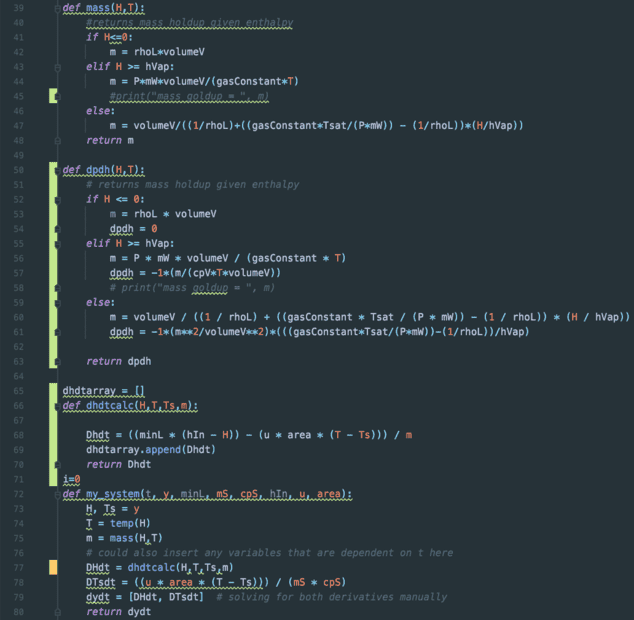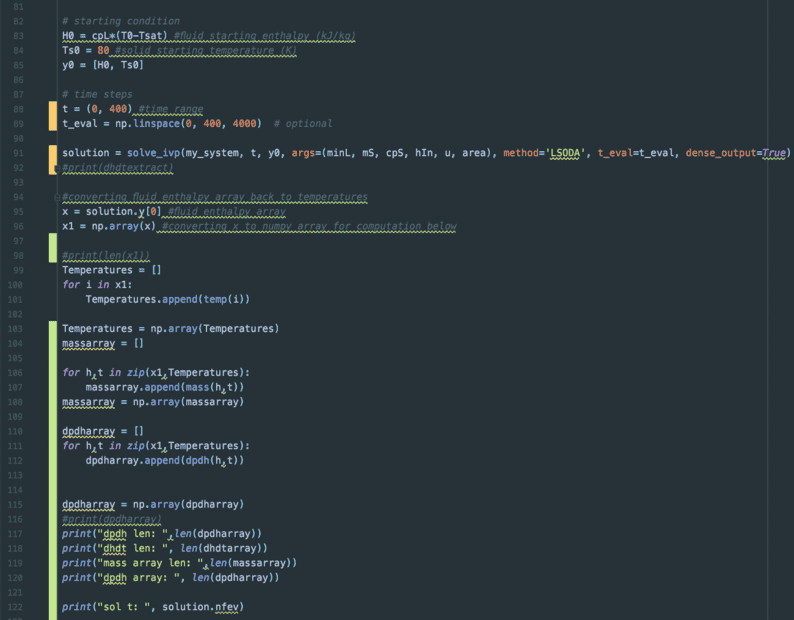Need a quick break now and will return to the code this evening

Chestermiller
Mentor
Yes you're right I should do this. It seems I can specify the time range and number of solution points (for h and t), however I can't specify the number of Dh/dt points that are calculated (seems bizarre but there are a number of forums on stack exchange explaining the same).

So yes I should do what you said.

Here is the code for reference:
View attachment 292991
View attachment 292992
View attachment 292993
Within your present approach, name a new subscripted variable and set that equal to dh/dt each time step.

Within your present approach, name a new subscripted variable and set that equal to dh/dt each time step.
Similar to what I've done between lines 99 and 112?

For some reason I went straight for extracting dh/dt directly from the my_system function. Maybe this wasn't a good approach

Chestermiller
Mentor
Similar to what I've done between lines 99 and 112?

For some reason I went straight for extracting dh/dt directly from the my_system function. Maybe this wasn't a good approach
I guess I'll stay out of this, and leave the coding to you.

I guess I'll stay out of this, and leave the coding to you.
I think your suggestion will work. Interesting how I was so focused on the first way I tried that I didn't attempt another.

Anyway I'll do this and post later this evening

I guess I'll stay out of this, and leave the coding to you.
Issue resolved. Just two questions:
1) How would you expect the mass flow out to vary over time?
2) I have a mass flow out array. I guess to convert to semi log I should do:
$$\dot{m}_{out, semilog}= \dot{m}_{j-1}-\dot{m}_{actual flow out}$$
Similar to the semi-log temperature plots?

Anyway this is the actual mass flow out versus time: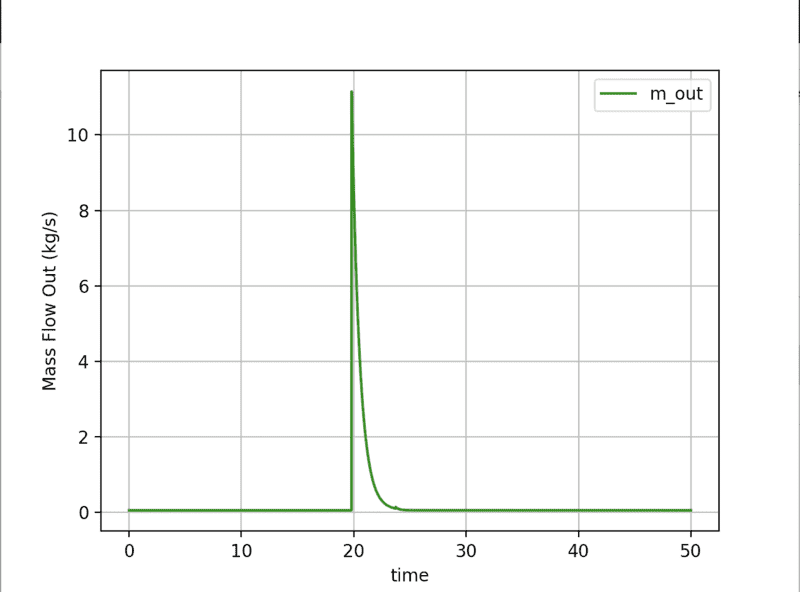I have yet to proofread my calculations (doing this now). This plot seems to say that the mass flow out is constant up until the point of phase change, when it rapidly increases. Once the phase change is complete then the mass flow out stabilises back at the initial value.

The semi-log plot would just be the mirror of this plot through the x axis I think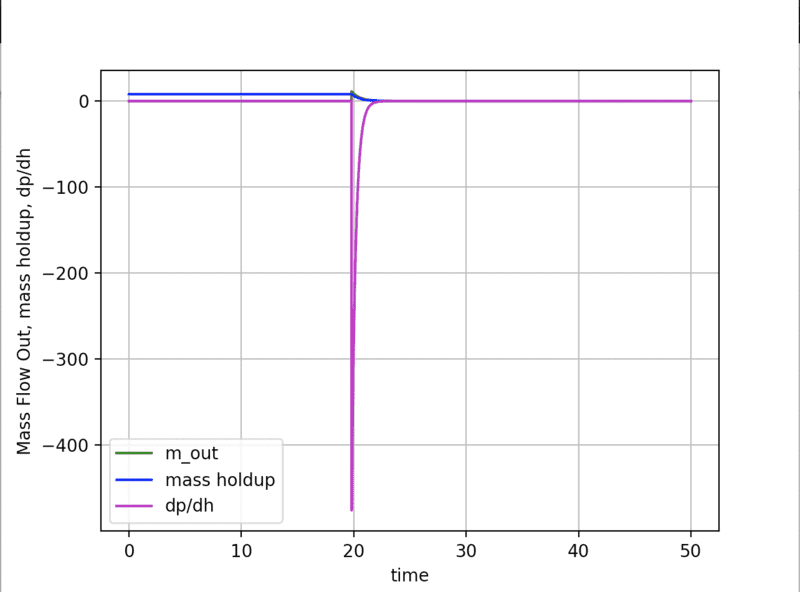Its a messy plot, but:
1) the blue line shows a constant mass holdup, a gradual decrease across the saturation zone (as liquid converts to vapour), and then a level out. I think this behaviour is expected
2) The purple line shows dp/dh = 0 in the liquid phase. As we move through the saturation zone dp/dh is negative, which I think is expected as density will decrease as enthalpy increases. Then it levels out after saturation as the change in density returns back to close to zero (not actually zero it reaches a minimum value of about -0.012 in this time range)

What I don't really understand is why the dp/dh value makes such a significant jump down at the beginning of saturation. I'm not sure if this is expected or not. Looking into this now

Last edited:
Chestermiller
Mentor
Issue resolved. Just two questions:
1) How would you expect the mass flow out to vary over time?
2) I have a mass flow out array. I guess to convert to semi log I should do:
$$\dot{m}_{out, semilog}= \dot{m}_{j-1}-\dot{m}_{actual flow out}$$
Similar to the semi-log temperature plots?
No. You just have your graphics software change the vertical axis to logarithmic.
Anyway this is the actual mass flow out versus time:
View attachment 293026
I have yet to proofread my calculations (doing this now). This plot seems to say that the mass flow out is constant up until the point of phase change, when it rapidly increases. Once the phase change is complete then the mass flow out stabilises back at the initial value.

The semi-log plot would just be the mirror of this plot through the x axis I think
Please have the software plot the horizontal axis from 15 seconds to 30 seconds only (i.e., expand the scale).

This is the way I expected the results to look.

Chestermiller
Mentor
View attachment 293028
Its a messy plot, but:
1) the blue line shows a constant mass holdup, a gradual decrease across the saturation zone (as liquid converts to vapour), and then a level out. I think this behaviour is expected
2) The purple line shows dp/dh = 0 in the liquid phase. As we move through the saturation zone dp/dh is negative, which I think is expected as density will decrease as enthalpy increases. Then it levels out after saturation as the change in density returns back to close to zero (not actually zero it reaches a minimum value of about -0.012 in this time range)

What I don't really understand is why the dp/dh value makes such a significant jump down at the beginning of saturation. I'm not sure if this is expected or not. Looking into this now
You are replacing high density liquid with a mixture of low density vapor and high density liquid. So the vapor is taking a large part of the volume, and room is being made for this.

I'm going to be unavailable for a few days because of the Thanksgiving holiday. Daughter's family from out-of-town is staying with us.

This is the way I expected the results to look.
0 to 30s (bit awkward to plot 15 to 30, I'll fix that today):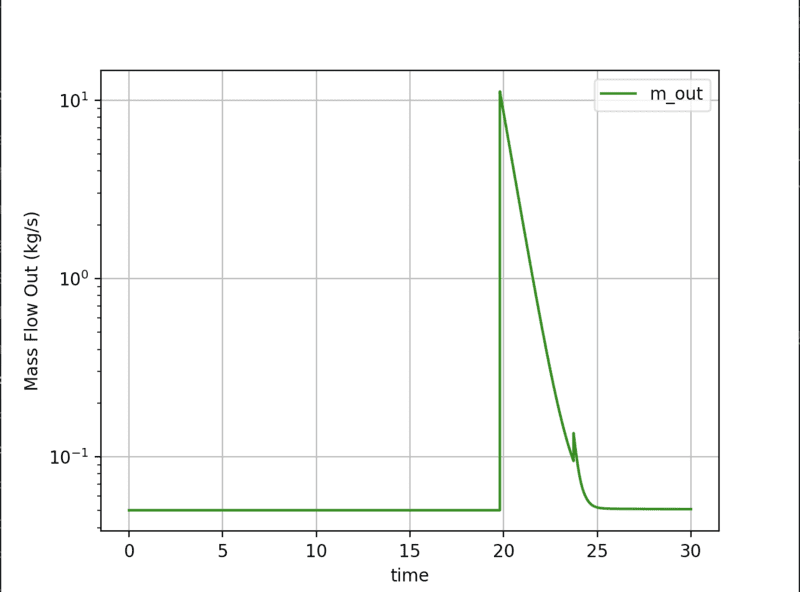You are replacing high density liquid with a mixture of low density vapor and high density liquid. So the vapor is taking a large part of the volume, and room is being made for this.
Understood
I'm going to be unavailable for a few days because of the Thanksgiving holiday. Daughter's family from out-of-town is staying with us.
Sounds great!If there's anything I can work on until next week I'd like to do that. I guess the model you described earlier is ready to go. If so, I can spend some time getting that right/cleaning the previous models

Thanks Chet and enjoy the holidays!

Last edited:
Chestermiller
Mentor
0 to 30s (bit awkward to plot 15 to 30, I'll fix that today):
View attachment 293061

Understood

Sounds great!If there's anything I can work on until next week I'd like to do that. I guess the model you described earlier is ready to go. If so, I can spend some time getting that right/cleaning the previous models

Thanks Chet and enjoy the holidays!
I'm back. What is the current status of the time- and spatial dependent model in your judgment?

I'm back. What is the current status of the time- and spatial dependent model in your judgment?
I was just about to comment.

I've spent yesterday evening and this evening summarising everything we've talked about into a powerpoint. Its about 90% done. I'll link it here via Google Drive or something tomorrow so anyone that might find this discussion useful in future can see it. It will now be easy to add in additional discussion to this as we go

I was actually going to ask you if the model you described in #127 ready to model or is there anything we haven't discussed in relation to this?

To answer my question - I've already got the mass flow out as a function of time part done (for the lumped parameter model as we previously talked about), so looking at your post #127 it seems good to go?

Chestermiller
Mentor
I was just about to comment.

I've spent yesterday evening and this evening summarising everything we've talked about into a powerpoint. Its about 90% done. I'll link it here via Google Drive or something tomorrow so anyone that might find this discussion useful in future can see it. It will now be easy to add in additional discussion to this as we go

I was actually going to ask you if the model you described in #127 ready to model or is there anything we haven't discussed in relation to this?

To answer my question - I've already got the mass flow out as a function of time part done (for the lumped parameter model as we previously talked about), so looking at your post #127 it seems good to go?
I think you are ready to start coding on the full model. Allow the dimension of the dependent variables, solution vector, etc to be variable so that you can readily change the number of tanks. Your early calculations should be done using only three tanks, which has all the features of a many tank model while allowing for easier diagnostics and debugging.

Your early calculations should be done using only three tanks, which has all the features of a many tank model while allowing for easier diagnostics and debugging.
Got it, and I agree, three tanks sounds like a good approach. I'll start on this first thing tomorrow. Great

The computational flow goes like this:
1. Focus first on the 1st tank
2. We know m˙0 because this the mass flow rate into the bed.
3. Use Eqn. 2 to get dh1/dt
4. Use Eqn. 4b to get dm1/dt
5. Use Eqn. 4a to get m˙1 (the mass flow rate from tank 1 into tank 2)
6. Use Eqn. 3 to get dTS,1/dt
Repeat steps 3-6 for each subsequent tank until we have the time derivatives of h in all the tanks. Eqns. 4 tell us the mass flow rate into each subsequent tank (for use in Eqn. 2).
Just one question on the above.

Taking tank #1, using the above computational flow:
We know ##m_0##, the flow into the bed. This is flow ##m_{j-1}## in equation 2
We also know ##h_0##, the enthalpy of the flow entering the bed, which is ##h_{j-1}##
Unknowns here are ##m_j## and ##h_j##.

Your point 2 above says use eq.2 to find ##\frac{dh_1}{dt}##

We can make the following replacement: ##m_j=\rho_j(V/n)##

So here is where my confusion is:
We need to somehow solve for ##\rho_j##, so that we can then get ##\frac{dh_1}{dt}##. We do have relations for ##\rho_j##. Which relation for ##\rho_j## be use is based on the enthalpy, which we do not have at point j.

So my question is - are we actually using the enthalpy at point ##j-1## to choose the density correlation at point ##j##?

Chestermiller
Mentor
Just one question on the above.

Taking tank #1, using the above computational flow:
We know ##m_0##, the flow into the bed. This is flow ##m_{j-1}## in equation 2
We also know ##h_0##, the enthalpy of the flow entering the bed, which is ##h_{j-1}##
Unknowns here are ##m_j## and ##h_j##.

Your point 2 above says use eq.2 to find ##\frac{dh_1}{dt}##

We can make the following replacement: ##m_j=\rho_j(V/n)##

So here is where my confusion is:
We need to somehow solve for ##\rho_j##, so that we can then get ##\frac{dh_1}{dt}##. We do have relations for ##\rho_j##. Which relation for ##\rho_j## be use is based on the enthalpy, which we do not have at point j.

So my question is - are we actually using the enthalpy at point ##j-1## to choose the density correlation at point ##j##?
We know these in all the tanks to begin with, because it is the initial condition. After that, they are known in all the tanks from the integration of the heat balance equation.

We know these in all the tanks to begin with, because it is the initial condition. After that, they are known in all the tanks from the integration of the heat balance equation.
Ah got it (really should have spotted that)

Actually a side question to this - I assume you've read a sizeable amount of books to get to the level of being able to answer such a wide range of questions on here, Stack Exchange, etc. I'd be curious to know what books stood out to you over the years? Not just thermo/ht related but anything at all under the umbrella of science/engineering

Working on the three tank model currently

Chestermiller
Mentor
Ah got it (really should have spotted that)

Actually a side question to this - I assume you've read a sizeable amount of books to get to the level of being able to answer such a wide range of questions on here, Stack Exchange, etc. I'd be curious to know what books stood out to you over the years? Not just thermo/ht related but anything at all under the umbrella of science/engineering

Working on the three tank model currently
I'll try to answer this in a little while. Meanwhile, I wanted to call something important to your attention.

Now that we have decided to use the trick of employing numerical dispersion to simulate the actual dispersion in the bed, it opens up many possibilities. In particular, the unwinding scheme that this approach entails does not even involve the parameters for the tank on the right. That means that only parameters for the present tank and the one to the left come into play. That means that , we can do the implicit time integration for each tank in the sequence, in turn from left to right (one-at-a time) without having to include implicitly all the tanks at once. So we are solving for two unknowns at a time, rather the 2n unknowns at a time. So first you find the new values at the end of the time step for the first tank, then for the 2nd tank, then for the third tank, etc. Much simpler to implement.

That means that , we can do the implicit time integration for each tank in the sequence, in turn from left to right (one-at-a time) without having to include implicitly all the tanks at once.
Understood
So we are solving for two unknowns at a time, rather the 2n unknowns at a time.
Understood also. So we can solve for all values in a tank, at all times, as long as we have the values in the tank to the left

Chestermiller
Mentor
Understood

Understood also. So we can solve for all values in a tank, at all times, as long as we have the values in the tank to the left
Presumably. But the preferred approach would be for each time step to involve a sweep through the bed from left to right, one tank at a time. Note that the existing one tank model can be used right now to simulate the first tank in the sequence for all times, and this can be used as a partial check on the full model.

Presumably. But the preferred approach would be for each time step to involve a sweep through the bed from left to right, one tank at a time. Note that the existing one tank model can be used right now to simulate the first tank in the sequence for all times, and this can be used as a partial check on the full model.
Back on the model now (I try take the evening off for exercise/being outdoors to keep the head clear). I think the 3 tank model is clear to me now. Theres just a bit of code adjusting yet to do

•Chestermiller
Presumably. But the preferred approach would be for each time step to involve a sweep through the bed from left to right, one tank at a time. Note that the existing one tank model can be used right now to simulate the first tank in the sequence for all times, and this can be used as a partial check on the full model.
Hi Chet, still working on sorting this out in my head.

Just one question. I am not exactly sure what I am assuming are the boundary/initial conditions, and what boundary/initial conditions are derived from these. It seems there are options for what parameters are assumed, and which are derived from the assumptions. So I'm wondering which parameters should I assume are known

For example:

Boundary conditions:
1)mass flow into the bed
2)temperature of the flow

Should I get the enthalpy from the temperature here?

And the initial conditions:
1) mass holdup
2) enthalpy of bed
3) temperature of bed
4) Temperature of fluid in bed
4) Density of bed

I guess the initial temperature of both the fluid and solid in the bed should be assumed here. Then the density, mass holdup, enthalpy can all be derived from this?

Chestermiller
Mentor
Hi Chet, still working on sorting this out in my head.

Just one question. I am not exactly sure what I am assuming are the boundary/initial conditions, and what boundary/initial conditions are derived from these. It seems there are options for what parameters are assumed, and which are derived from the assumptions. So I'm wondering which parameters should I assume are known

For example:

Boundary conditions:
1)mass flow into the bed
2)temperature of the flow

Should I get the enthalpy from the temperature here?
Sure
And the initial conditions:
1) mass holdup
2) enthalpy of bed
3) temperature of bed
4) Temperature of fluid in bed
4) Density of bed

I guess the initial temperature of both the fluid and solid in the bed should be assumed here. Then the density, mass holdup, enthalpy can all be derived from this?
Do exactly the same thing you did for the 1 tank model.

Sure

Do exactly the same thing you did for the 1 tank model.
For that model I assumed H0 and Ts0 in the tank, so I found m, t and rho from H0.

Will do

Chestermiller
Mentor
For that model I assumed H0 and Ts0 in the tank, so I found m, t and rho from H0.

Will do
You are assuming that the bed temperature and the temperature of the fluid in the bed are initially the same, right?